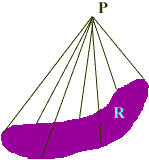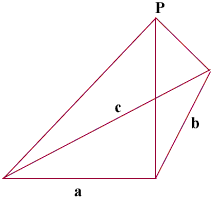Quandaries and Queries Secondary Student How do I calculate the volume of an irregular shaped tetrahedron where:- side a = 1.4 m side b = 1.4 m side c = 1.2 m and height = 0.75 m at the junction of sides a and b. Thank you in anticipation Peter Hi Peter, The volume follows from a result that says if you take some region R in the plane with an area, some point P not in the plane and form a cone by joining every point on the boundary of R to P with a straight line then the volume of the cone is  1/3(the area of the base)(the height).By your description you have a tetrahedron with a base triangle having sides of lengths a, b and c and a vertex P which is 0.75 m above the plane containing the base triangle.The volume of the tetrahedron is then 1/3(the area of the base triangle)0.75 m3 The area of the base triangle can be found using Heron's Formula. Penny Go to Math Central